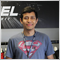# Array help30

Hello, could you help me?

The "GetPrice" function captures prices on objects, but I'm not able to store them in the "taxas[]" array.

Can anyone help me?

```#property copyright "Copyright 2019"
#property version   "1.00"
#property strict
#property indicator_chart_window
//---
long chartID;
int windowNo;
double taxas;
//+------------------------------------------------------------------+
//| Custom indicator initialization function                         |
//+------------------------------------------------------------------+
int OnInit()
{
chartID=ChartID();
windowNo=ChartWindowFind();
//---
return(INIT_SUCCEEDED);
}
//+------------------------------------------------------------------+
//| Custom indicator iteration function                              |
//+------------------------------------------------------------------+
int OnCalculate(const int rates_total,
const int prev_calculated,
const datetime &time[],
const double &open[],
const double &high[],
const double &low[],
const double &close[],
const long &tick_volume[],
const long &volume[],
{
//---

//--- return value of prev_calculated for next call
return(rates_total);
}
//+------------------------------------------------------------------+
//|                                                                  |
//+------------------------------------------------------------------+
void  OnChartEvent(const int id,
const long& lparam,
const double& dparam,
const string& sparam)
{
if(id==CHARTEVENT_OBJECT_CLICK)
GetPrice(taxas);

for(int i=0; i<50; i++) {
printf(taxas[i]);
}
//---
}
//+------------------------------------------------------------------+
//|                                                                  |
//+------------------------------------------------------------------+
void GetPrice(double &taxas[])
{
string name;
int total=ObjectsTotal(chartID,windowNo);
for(int i=0; i<total; i++)
{
if(ObjectType((name=ObjectName(i)))==OBJ_RECTANGLE && StringFind(name,"Verified")!=-1)
{
taxas[i] = (ObjectGetDouble(chartID,name,OBJPROP_PRICE1));
}
}
}
//+------------------------------------------------------------------+
```1754

Hello,

Just try double Array[];30

Nelson Silva:

Hello,

Just try double Array[];

2019.09.09 21:29:53.749 GetRectPrice EURUSD,M5: array out of range in 'GetRectPrice.mq4' (56,22)1754

Danillo Souza:
2019.09.09 21:29:53.749 GetRectPrice EURUSD,M5: array out of range in 'GetRectPrice.mq4' (56,22)

Hi, Danilo

Before you scan for the array elements you should get the ArraySize(), then you limit the scan total elements in the FOR block.

```      int ArrayTotal = ArraySize(taxas);

for(int i=0; i<=ArrayTotal; i++) {
printf(taxas[i]);
}

```30

Nelson Silva:

Hi, Danilo

Before you scan for the array elements you should get the ArraySize(), then you limit the scan total elements in the FOR block.

Like this?

```if(id==CHARTEVENT_OBJECT_CLICK)
GetPrice(taxas);

int ArrayTotal = ArraySize(taxas);

for(int i = 0; i < ArrayTotal; i++) {
printf(taxas[i]);
}```1754

Yes, with this code you simply avoid the arrayOutOfRange error return.